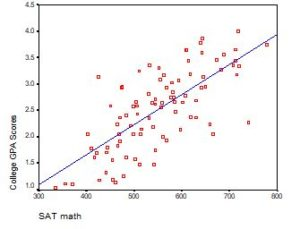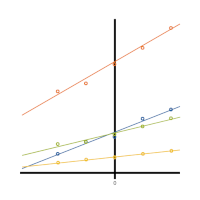# centering

### Concepts in Linear Regression to know before learning Multilevel Models

November 21st, 2023 by

It’s a very common analysis to need to use. I have to say, learning it is not so easy on your own. The concepts of random effects are hard to wrap your head around and there is a ton of new vocabulary and notation. Sadly, this vocabulary and notation is not consistent across articles, books, and software, so you end up having to do a lot of translating.

### Interpreting the Intercept in a Regression Model

February 21st, 2023 by

Interpreting the Intercept in a regression model isn’t always as straightforward as it looks.

Here’s the definition: the intercept (often labeled the constant) is the expected value of Y when all X=0. But that definition isn’t always helpful. So what does it really mean?

### Regression with One Predictor XIf X sometimes equals 0, the intercept is simply the expected value of Y at that value. In other words, it’s the mean of Y at one value of X. That’s meaningful.

If X never equals 0, then the intercept has no intrinsic meaning. You literally can’t interpret it. That’s actually fine, though. You still need that intercept to give you unbiased estimates of the slope and to calculate accurate predicted values. So while the intercept has a purpose, it’s not meaningful.

Both these scenarios are common in real data. (more…)

### Member Training: Centering

November 30th, 2022 byCentering variables is common practice in some areas, and rarely seen in others. That being the case, it isn’t always clear what are the reasons for centering variables.Is it only a matter of preference, or does centering variables help with analysis and interpretation? (more…)

### Centering a Covariate to Improve Interpretability

April 9th, 2021 by

Centering a covariate –a continuous predictor variable–can make regression coefficients much more interpretable. That’s a big advantage, particularly when you have many coefficients to interpret. Or when you’ve included terms that are tricky to interpret, like interactions or quadratic terms.

For example, say you had one categorical predictor with 4 categories and one continuous covariate, plus an interaction between them.

First, you’ll notice that if you center your covariate at the mean, there is (more…)

### Member Training: Model Building Approaches

January 1st, 2019 by

There is a bit of art and experience to model building. You need to build a model to answer your research question but how do you build a statistical model when there are no instructions in the box?

Should you start with all your predictors or look at each one separately? Do you always take out non-significant variables and do you always leave in significant ones?

### Should You Always Center a Predictor on the Mean?

December 2nd, 2011 by

Centering predictor variables is one of those simple but extremely useful practices that is easily overlooked.

It’s almost too simple.

Centering simply means subtracting a constant from every value of a variable.  What it does is redefine the 0 point for that predictor to be whatever value you subtracted.  It shifts the scale over, but retains the units.

The effect is that the slope between that predictor and the response variable doesn’t (more…)